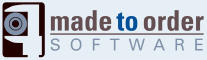# compute

## XOr

SWF Action
Action Category:
Logical and Bitwise
Action Details:
0
Action Identifier:
98
Action Structure:
<n.a.>
Action Length:
0 byte(s)
Action Stack:
pop 2 (i), push 1 (i)
Action Operation:
i1 := pop();
i2 := pop();
r := i2 ^ i1;
push(r);
Action Flash Version:
5

Pop two integers, compute the bitwise XOR and push the result back on the stack.

This operator is used to generate a bitwise NOT with an immediate value of -1. (There is not bitwise NOT action.)

## String Equal

SWF Action
Action Category:
Comparisons
Action Details:
0
Action Identifier:
19
Action Structure:
<n.a.>
Action Length:
0 byte(s)
Action Stack:
pop 2 (s), push 1 (b)
Action Operation:
s1 := pop();
s2 := pop();
r := s2 == s1;
push(r);
Action Flash Version:
4

Pops two strings, compute the equality and put the Boolean result back on the stack.

IMPORTANT

The true meaning of this operator was to apply the String cast to both values, then compare the result as strings. This is not really good JavaScript as per ECMA, so later Macromedia added the strict comparison operators instead. This is why this action should only be used in a Version 4 of SWF. Newer versions should use Strict Equal or plain Equal.

## Ord (multi-byte)

SWF Action
Action Category:
String and Characters
Action Details:
(multi-byte)
Action Identifier:
54
Action Structure:
<n.a.>
Action Length:
0 byte(s)
Action Stack:
pop 1 (s), push 1 (i)
Action Operation:
s1 := pop();
r := s1;
push(r);
Action Flash Version:
4

Pops one string, compute the multi-byte value of its first character and put it on the stack.

In Flash, multi-byte characters are limited to 16 bits (UCS-2).

## Ord

SWF Action
Action Category:
String and Characters
Action Details:
0
Action Identifier:
50
Action Structure:
<n.a.>
Action Length:
0 byte(s)
Action Stack:
pop 1 (s), push 1 (i)
Action Operation:
s1 := pop();
r = s1;
push(r);
Action Flash Version:
4

Pop one string, compute the ASCII value of its first character and put it back on the stack.

This function does not take UTF-8 in account. In other words, it can be used to parse a string byte per byte. To get the UTF-8 value of characters, use the Ord (multi-byte) instead.

## Or

SWF Action
Action Category:
Logical and Bitwise
Action Details:
0
Action Identifier:
97
Action Structure:
<n.a.>
Action Length:
0 byte(s)
Action Stack:
pop 2 (i), push 1 (i)
Action Operation:
i1 := pop();
i2 := pop();
r := i2 | i1;
push(r);
Action Flash Version:
5

Pop two integers, compute the bitwise OR and push the result back on the stack.

## Modulo

SWF Action
Action Category:
Arithmetic
Action Details:
0
Action Identifier:
63
Action Structure:
<n.a.>
Action Length:
0 byte(s)
Action Stack:
pop 2 (n), push 1 (n)
Action Operation:
n1 := pop();
n2 := pop();
if(is_integer(n1) && is_integer(n2)) {
r := i2 % i1;
}
else {
r := f2 % f1;   // in C it would be fmod(f2, f1)
}
push(r);
Action Flash Version:
5

Pop two numbers, compute the modulo and push the result back on the stack.

Note that the operator can compute a floating point modulo (in case at least one of the parameters is a floating point.)

## Logical Or

SWF Action
Action Category:
Logical and Bitwise
Action Details:
0
Action Identifier:
17
Action Structure:
<n.a.>
Action Length:
0 byte(s)
Action Stack:
pop 2 (b), push 1 (b)
Action Operation:
b1 := pop();
b2 := pop();
r := b2 || b1;
push(r);
Action Flash Version:
4

Pop two values, compute the Logical OR and put the Boolean result back on the stack.

## Logical Not

SWF Action
Action Category:
Logical and Bitwise
Action Details:
0
Action Identifier:
18
Action Structure:
<n.a.>
Action Length:
0 byte(s)
Action Stack:
pop 1 (b), push 1 (b)
Action Operation:
b1 := pop();
r := ! b1;
push(r);
Action Flash Version:
4

Pop one value, compute the Logical NOT and put the result back on the stack.

## Logical And

SWF Action
Action Category:
Logical and Bitwise
Action Details:
0
Action Identifier:
16
Action Structure:
<n.a.>
Action Length:
0 byte(s)
Action Stack:
pop 2 (b), push 1 (b)
Action Operation:
b1 := pop();
b2 := pop();
r := b2 && b1;
push(r);
Action Flash Version:
4

Pop two values, compute the Logical AND and put the Boolean result back on the stack.

## Less Than (typed)

SWF Action
Action Category:
Comparisons
Action Details:
(typed)
Action Identifier:
72
Action Structure:
<n.a.>
Action Length:
0 byte(s)
Action Stack:
pop 2 (a), push 1 (b)
Action Operation:
a1 := pop();
a2 := pop();
if(is_int(a1) && is_int(a2)) {
i1 := (int)a1;
i2 := (int)a2;
r := i2 < i1;    // compare integers
}
else if(is_numeric(a1) && is_numeric(a2)) {
f1 := (float)a1;
f2 := (float)a2;
r := f2 < f1;    // compare floating points
}
else {
s1 := (string)a1;
s2 := (string)a2;
r := s2 < s1;    // compare characters, case sensitive
}
push(r);
Action Flash Version:
5